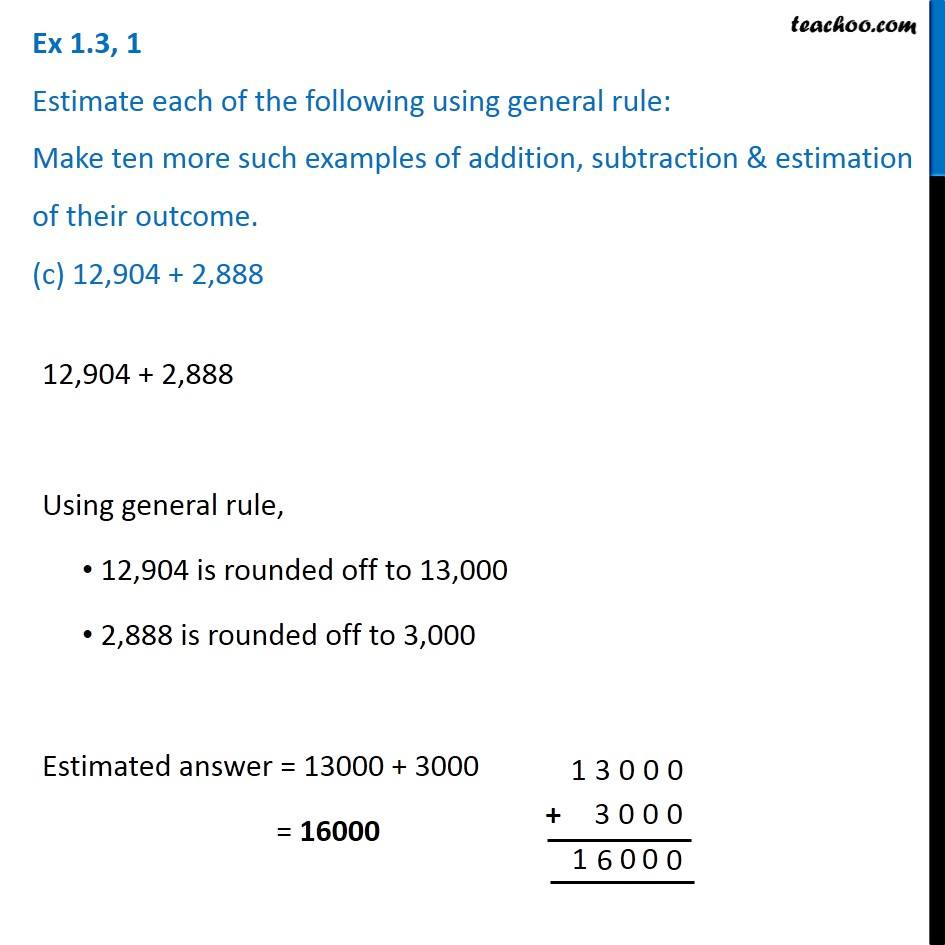1. Chapter 1 Class 6 Knowing our Numbers
2. Serial order wise
3. Ex 1.3

Transcript

Ex 1.3, 1 Estimate each of the following using general rule: Make ten more such examples of addition, subtraction & estimation of their outcome. (c) 12,904 + 2,88812,904 + 2,888 Using general rule, 12,904 is rounded off to 13,000 2,888 is rounded off to 3,000 Estimated answer = 13000 + 3000 = 16000